# Thermodynamic relations

(diff) ← Older revision | Latest revision (diff) | Newer revision → (diff)

Notation used:

•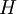$H$ is the enthalpy
•$T$ is the temperature
•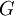$G$ is the Gibbs energy function
•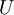$U$ is the internal energy,
•$V$ is the volume
•$A$ is the Helmholtz energy function
•$p$ is the pressure
•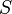$S$ is the entropy

These thermodynamic relations are also known as coefficient relations.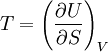$T=\left(\frac{\partial U}{\partial S}\right)_V$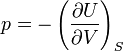$p=-\left(\frac{\partial U}{\partial V}\right)_S$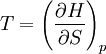$T=\left(\frac{\partial H}{\partial S}\right)_p$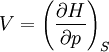$V=\left(\frac{\partial H}{\partial p}\right)_S$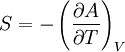$S=-\left(\frac{\partial A}{\partial T}\right)_V$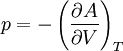$p=-\left(\frac{\partial A}{\partial V}\right)_T$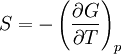$S=-\left(\frac{\partial G}{\partial T}\right)_p$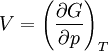$V=\left(\frac{\partial G}{\partial p}\right)_T$